# How to Calculate and Solve for Permeability Number | Refractories

The permeability number is illustrated by the image below.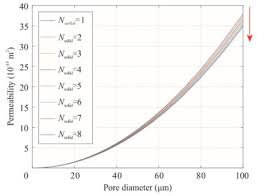To compute for the permeability number, five essential parameters are needed and these parameters are Volume of Air (V), Height of the Specimen (H), Cross-sectional Area (A), Air Pressure (P) and Time (t).

The formula for calculating permeability number:

PN = VH/APt

Where:

PN = Permeability Number
V = Volume of Air
H = Height of the Specimen
A = Cross-sectional Area
P = Air Pressure
t = Time

Let’s solve an example;
Find the permeability number when the volume of air is 2, the height of the specimen is 7, the cross-sectional area is 3, the air pressure is 9 and the time is 4.

This implies that;

V = Volume of Air = 2
H = Height of the Specimen = 7
A = Cross-sectional Area = 3
P = Air Pressure = 9
t = Time = 4

PN = VH/APt
PN = (2)(7)/(3)(9)(4)
PN = (14)/(108)
PN = 0.129

Therefore, the permeability number is 0.129.

Nickzom Calculator – The Calculator Encyclopedia is capable of calculating the permeability number.

To get the answer and workings of the permeability number using the Nickzom Calculator – The Calculator Encyclopedia. First, you need to obtain the app.

You can get this app via any of these means:

You can also try the demo version via https://www.nickzom.org/calculator

Apple (Paid) – https://itunes.apple.com/us/app/nickzom-calculator/id1331162702?mt=8
Once, you have obtained the calculator encyclopedia app, proceed to the Calculator Map, then click on Materials and Metallurgical under Engineering.Now, Click on Refractories under Materials and Metallurgical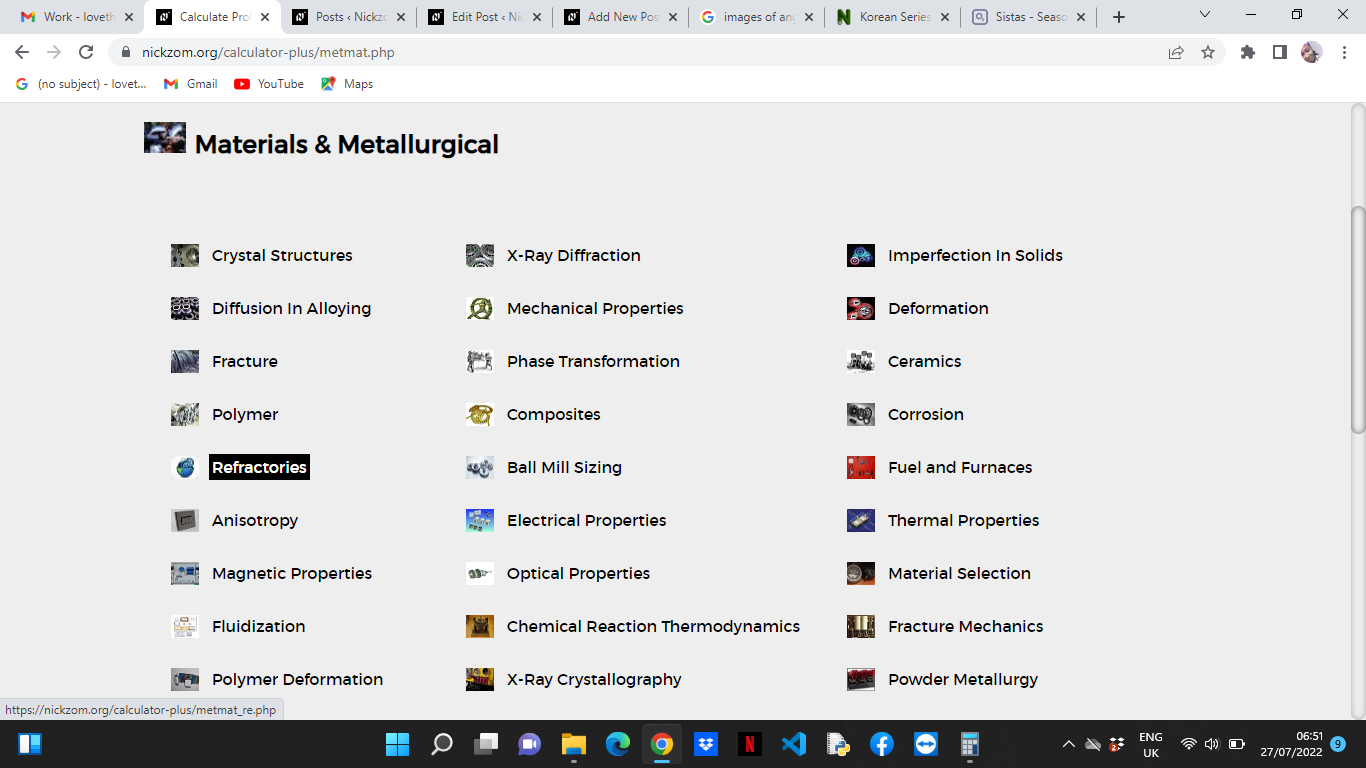Now, Click on Permeability Number under Refractories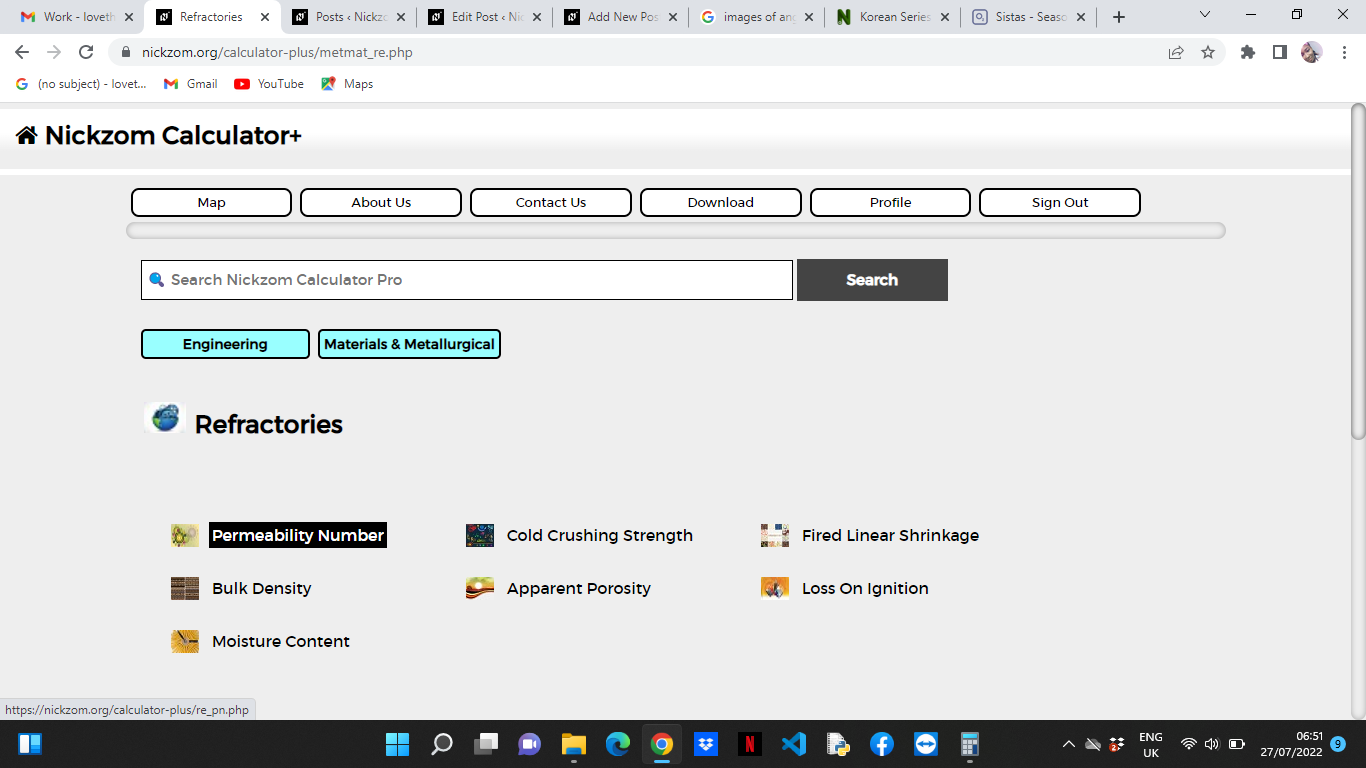The screenshot below displays the page or activity to enter your values, to get the answer for the permeability number according to the respective parameter which is the Volume of Air (V), Height of the Specimen (H), Cross-sectional Area (A), Air Pressure (P) and Time (t).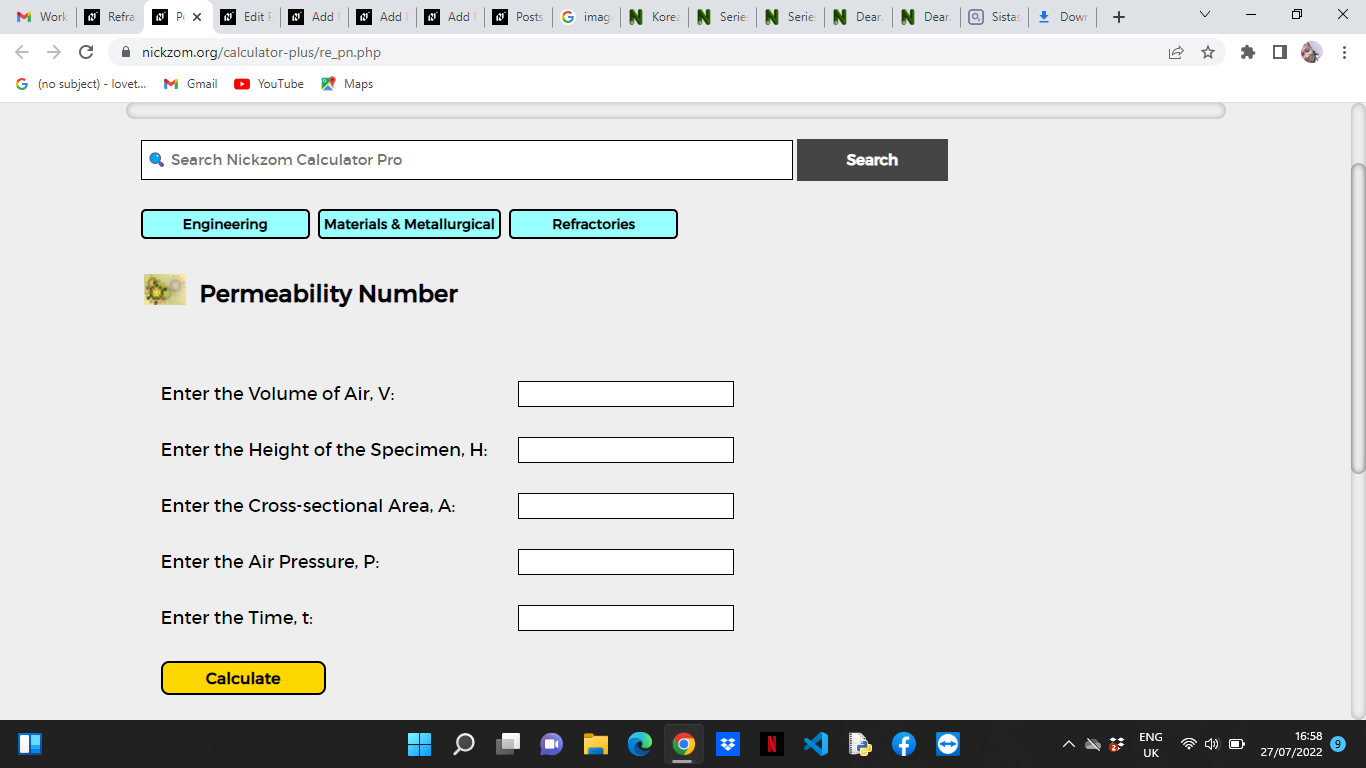Now, enter the values appropriately and accordingly for the parameters as required by the Volume of Air (V) is 2, Height of the Specimen (H) is 7, Cross-sectional Area (A) is 3, Air Pressure (P) is 9 and Time (t) is 4.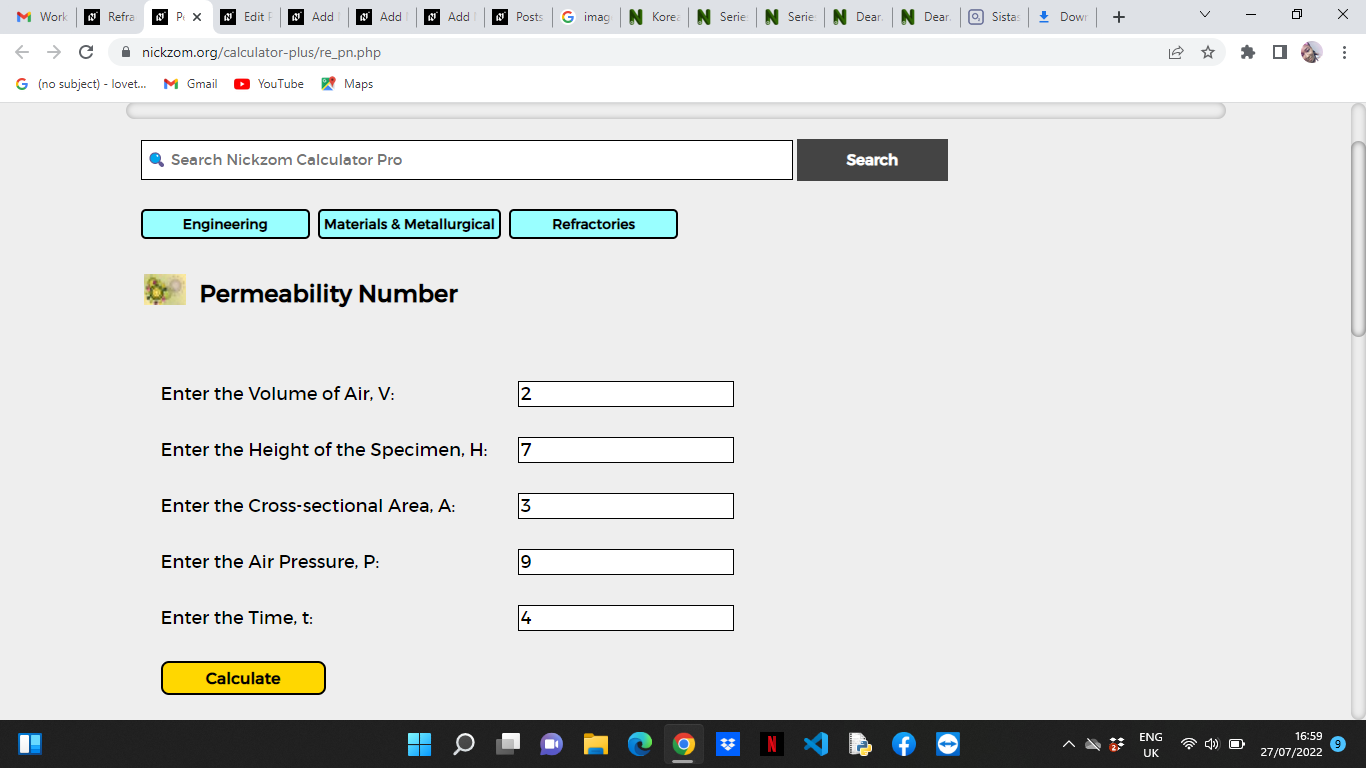Finally, Click on Calculate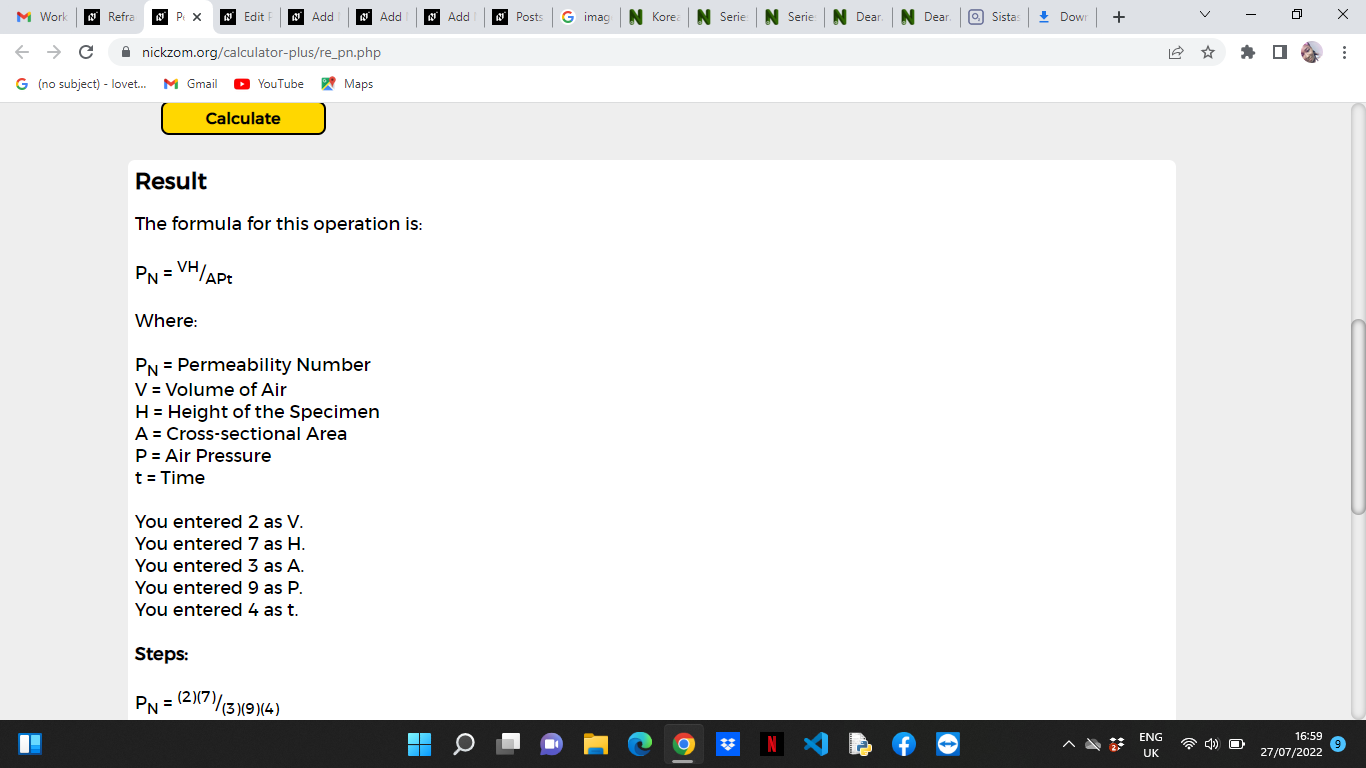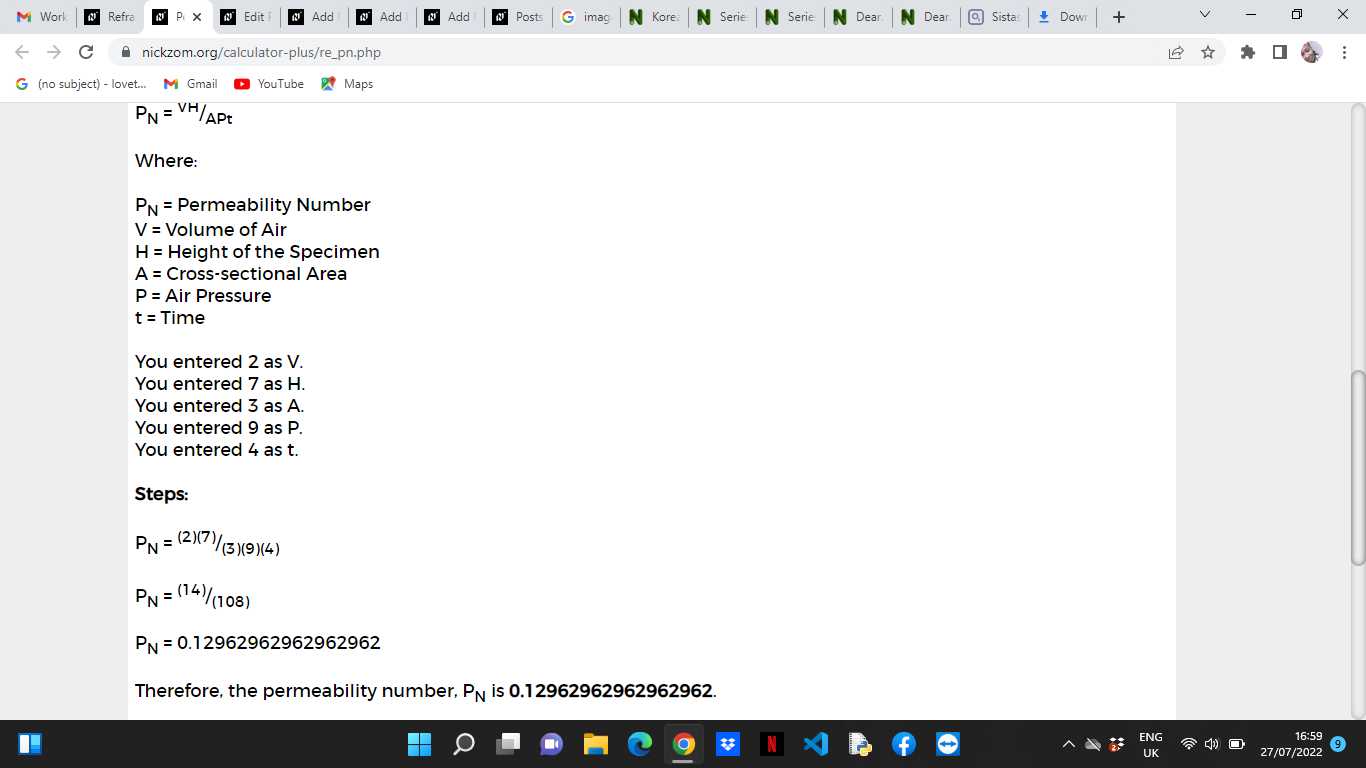As you can see from the screenshot above, Nickzom Calculator– The Calculator Encyclopedia solves for the permeability number and presents the formula, workings and steps too.